# Simple circuits enable oscilloscope art

Russ Williams

EDN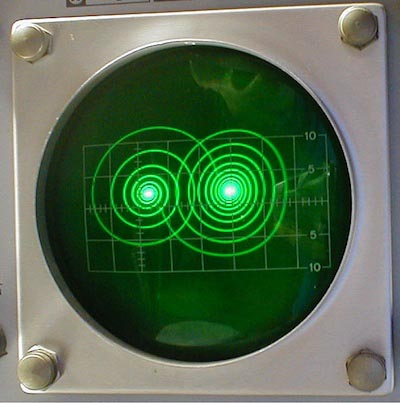Figure 1. Display of R-L-C circuit.

There is one analog graphic which remains impressive in spite of all the computer graphics programs available for screen savers and such (Figure 1). Known as quadrature art, the display can be produced with an oscilloscope, a variable frequency square wave generator, and an R-L-C resonant circuit (Figure 2). This can be used to entertain young and nontechnical visitors to the lab. The most common manifestation of the display is two spirals. What do you think they look like?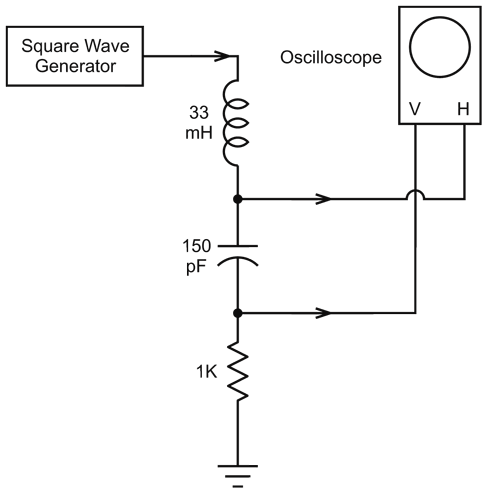Figure 2. R-L-C circuit to produce double spiral display.

The frequency of the square wave is about one tenth the resonant frequency of the R-L-C circuit. When the frequency of the square wave generator is increased, the double spiral unwinds until it becomes a single circle. The oscilloscope is driven at its horizontal and vertical inputs and the sweep circuit is not used. Since we are working with audio frequencies, a cheap oscilloscope will do fine.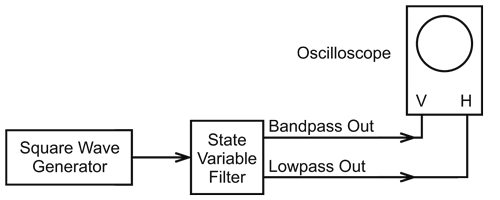Figure 3. State variable filter to obtain double spiral display.

A more versatile version of the display can be had by using a state variable filter instead of the R-L-C circuit. As shown in Figure 3, the vertical plates of the oscilloscope are attached to the bandpass (BP) output and the horizontal plates are attached to the lowpass (LP) output of the filter. Figure 4 shows the circuit of the state variable filter. Figure 5 shows the display produced by this setup.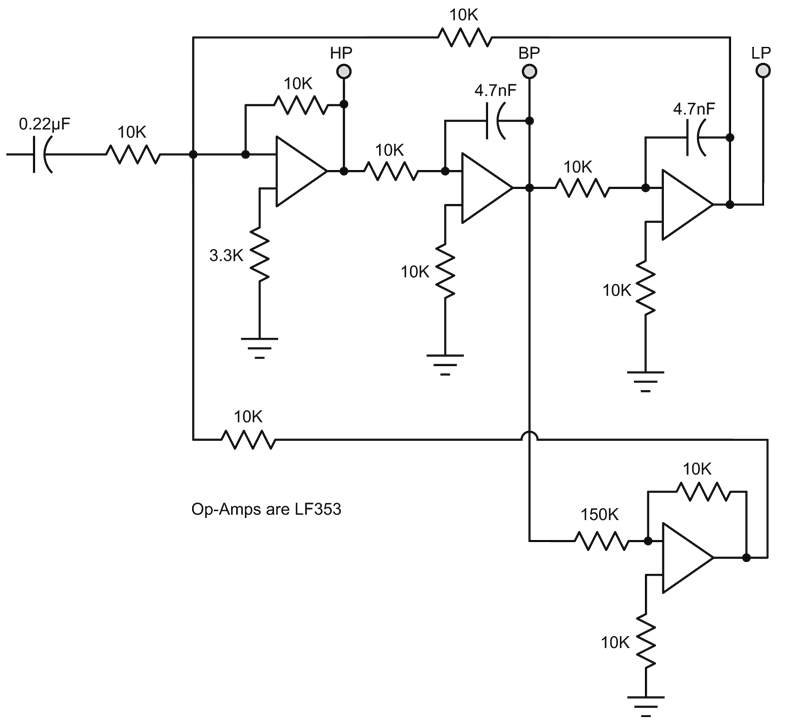Figure 4. The state variable filter.

As shown in Figure 6, different displays can be produced by using different input waveforms. For instance, a short pulse instead of the square wave will produce a single spiral. A triangular waveform input will produce a looping display. Using the highpass (HP) output of the filter instead of the lowpass or bandpass will produce different kinds of displays.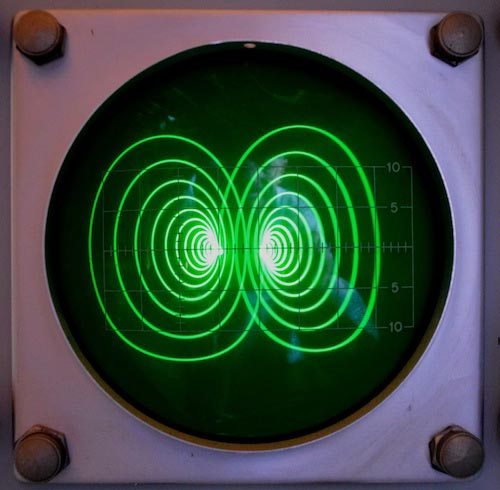Figure 5. Display of filter.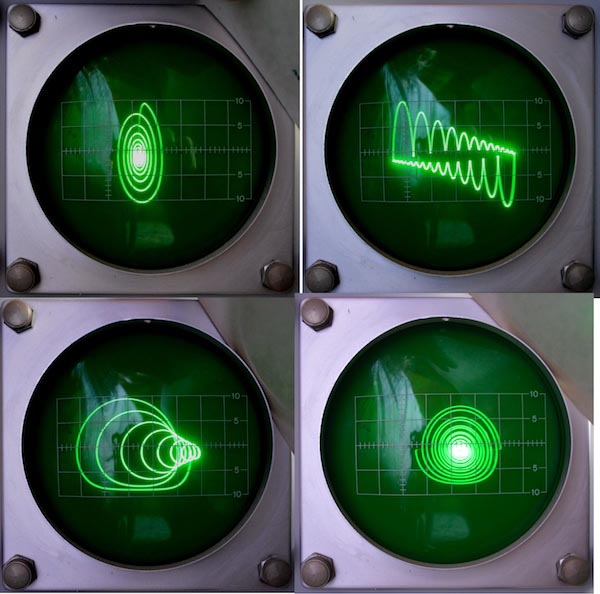Figure 6. Other waveforms obtainable with state variable filter.

References:

1. Lancaster, Don. The Active-Filter Cookbook, pp. 219-222